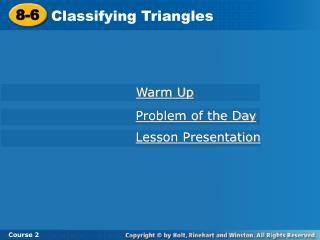DownloadDownload Presentation8-6

# 8-6

Télécharger la présentation## 8-6

- - - - - - - - - - - - - - - - - - - - - - - - - - - E N D - - - - - - - - - - - - - - - - - - - - - - - - - - -
##### Presentation Transcript

1. 8-6 Classifying Triangles Course 2 Warm Up Problem of the Day Lesson Presentation

2. 8-6 Classifying Triangles Course 2 Warm Up 1.What is the name of a six-sided figure? 2. What is the definition of an acute angle? 3. The measure of one of two supplementary angles is 32°. What is the measure of the other angle? hexagon an angle that measures less than 90° 148°

3. 8-6 Classifying Triangles Course 2 Problem of the Day Use 6 congruent line segments to form 4 equilateral triangles. (Hint: Use toothpicks or other objects to make a three-dimensional model with the segments.) Place the segments so they form a pyramid.

4. 8-6 Classifying Triangles Course 2 Learn to classify triangles by their side lengths and angle measures.

5. 8-6 Classifying Triangles Course 2 Insert Lesson Title Here Vocabulary scalene triangle isosceles triangle equilateral triangle acute triangle obtuse triangle right triangle

6. 8-6 Classifying Triangles Course 2 One way to classify triangles is by the lengths of their sides. Triangles classified by sides A scalene triangle has no congruent sides.

7. 8-6 Classifying Triangles Course 2 One way to classify triangles is by the lengths of their sides. Triangles classified by sides An isosceles triangle has at least 2 congruent sides

8. 8-6 Classifying Triangles Course 2 One way to classify triangles is by the lengths of their sides. Triangles classified by sides In an equilateral triangle, all of the sides are congruent.

9. 8-6 Classifying Triangles Course 2 One way to classify triangles is by the lengths of their sides. Another way is by the measures of their angles. Triangles classified by angles In an acute triangle, all of the angles are acute.

10. 8-6 Classifying Triangles Course 2 One way to classify triangles is by the lengths of their sides. Another way is by the measures of their angles. Triangles classified by angles An obtuse trianglehas one obtuse angle.

11. 8-6 Classifying Triangles Course 2 One way to classify triangles is by the lengths of their sides. Another way is by the measures of their angles. Triangles classified by angles A right triangle has one right angle.

12. 8-6 Classifying Triangles Course 2 Additional Example 1: Classifying Triangles Classify each triangle according to its sides and angles. Two congruent sides A. isosceles acute Three acute angles This is an isosceles acute triangle. B. scalene No congruent sides right One right angle This is a scalene right triangle.

13. 8-6 Classifying Triangles Course 2 Additional Example 1: Classifying Triangles Classify each triangle according to its sides and angles. equilateral Three congruent sides C. acute Three acute angles This is a equilateral acute triangle. D. scalene No congruent sides right One right angle This is a scalene right triangle.

14. 8-6 Classifying Triangles Course 2 Insert Lesson Title Here Check It Out: Example 1 Classify each triangle according to its sides and angles. isosceles Two congruent sides A. obtuse One obtuse angle This is an isosceles obtuse triangle. Two congruent sides B. isosceles right One right angle This is an isosceles right triangle.

15. 8-6 Classifying Triangles Course 2 Insert Lesson Title Here Check It Out: Example 1 Classify each triangle according to its sides and angles. scalene No congruent sides C. obtuse One angle exceeding 90° This is a scalene obtuse triangle. scalene D. No congruent sides obtuse One angle exceeding 90° This is a scalene obtuse triangle.

16. 8-6 Classifying Triangles Course 2 Additional Example 2: Identifying Triangles Identify the different types of triangles in the figure, and determine how many of each there are. 2 Scalene ABD, BCD 2 Isosceles ACE, ABC Right 2 ABD, BCD 1 Obtuse ACE 1 Acute ABC

17. 8-6 Classifying Triangles DJA, JDG HJI, BCD DFE DJA, ADG JDG, JHI, BCD, DEF Course 2 Insert Lesson Title Here Check It Out: Example 2 Identify the different types of triangles in the figure, and determine how many of each there are. A B I Type How many Name J C D H Isosceles 5 F G E 2 Obtuse Acute 4

18. 8-6 Classifying Triangles Course 2 Insert Lesson Title Here Lesson Quiz Classify each triangle according to its sides and angles. 1. 7ft, 8ft, 12ft 2. 45°, 90°, 45° 3. 12, 9, 12 4. 120°, 20°, 40° 5. Identify the different types of triangles in the figure and determine how many of each there are. scalene right isosceles obtuse 1 isosceles acute triangle, 1 equilateral triangle, 1 scalene right triangle